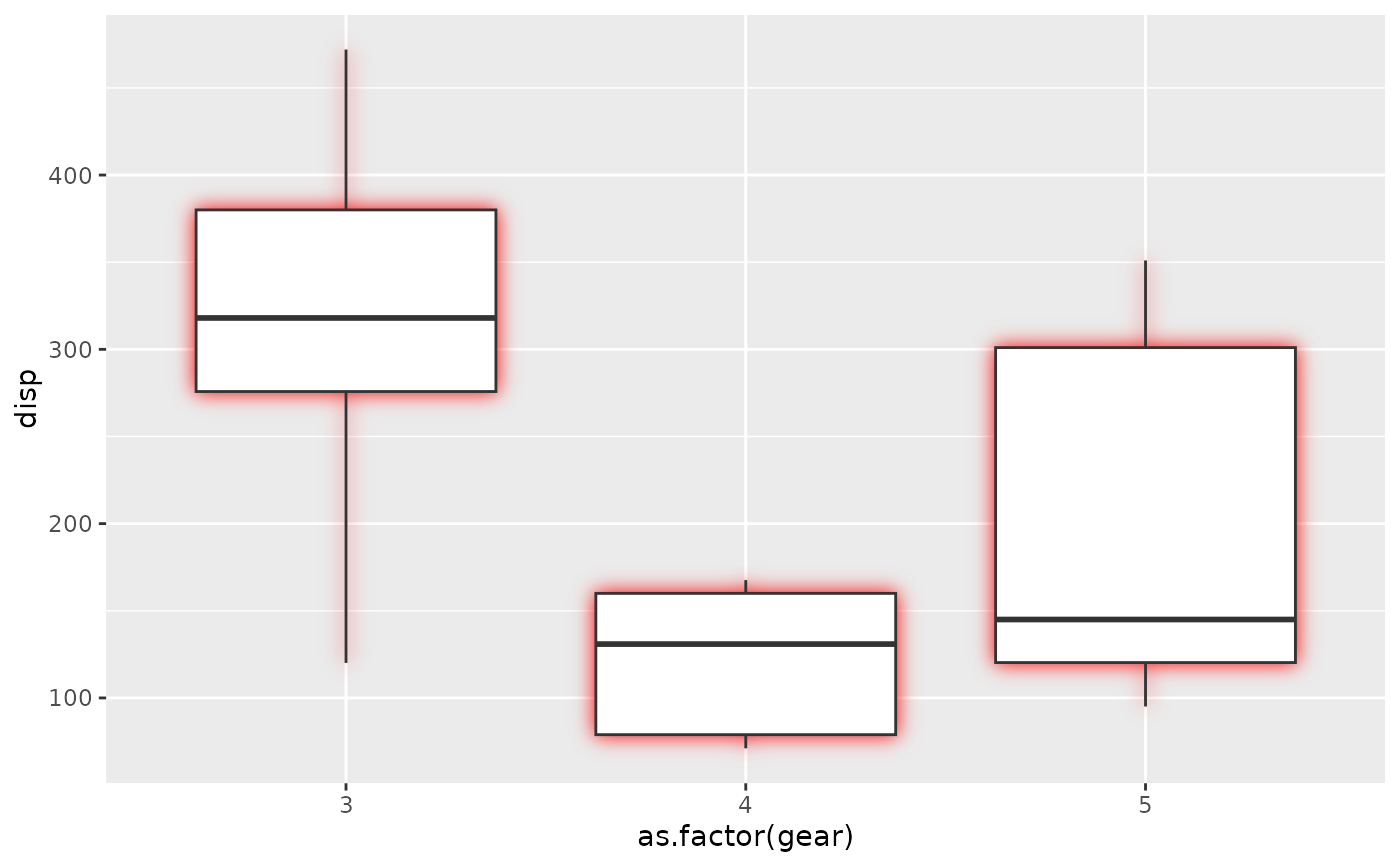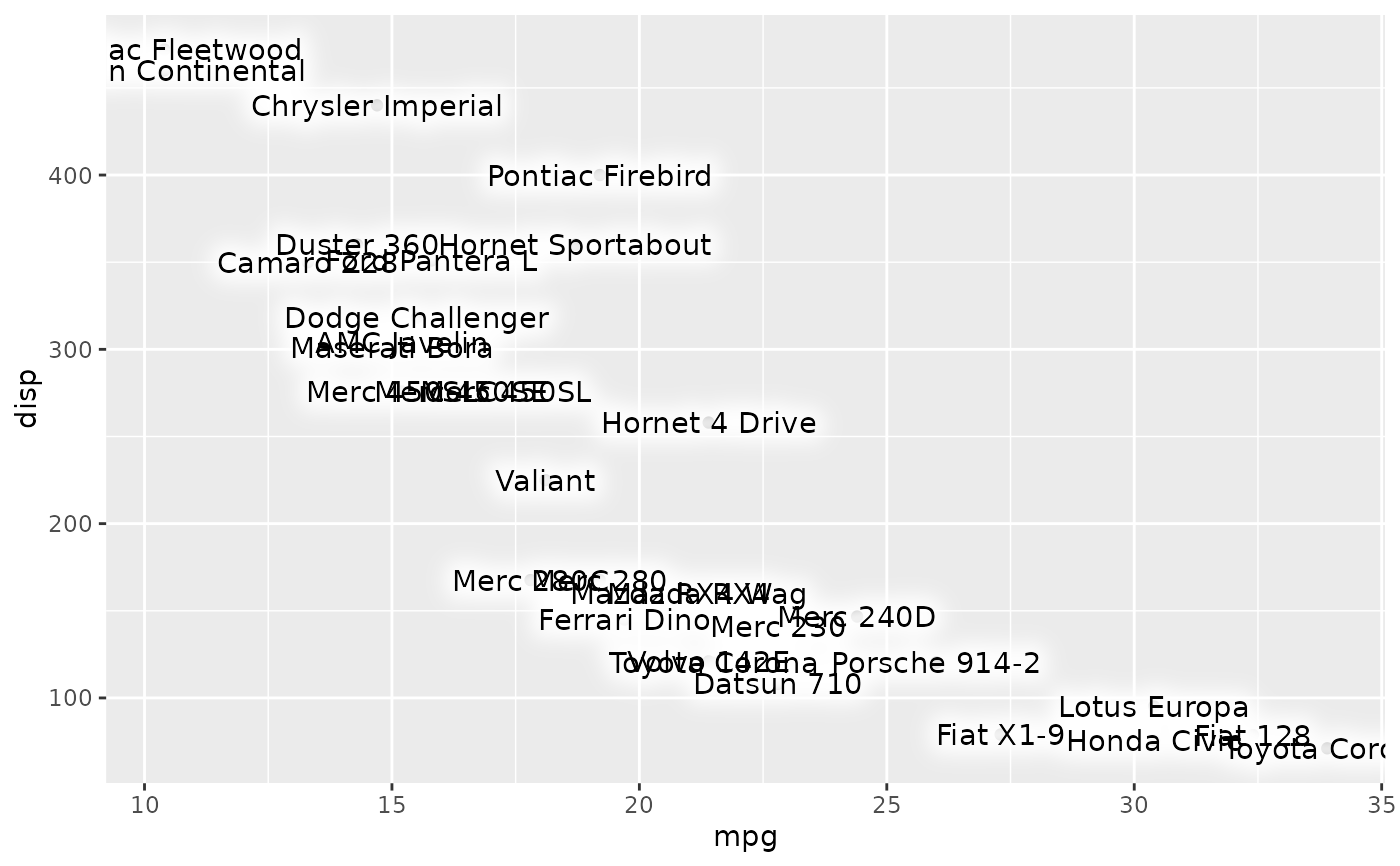This filter adds an outer glow to your layer with a specific colour and size. For very thin objects such as text it may be beneficial to add some expansion. See the examples for this.

with_outer_glow(x, colour = "black", sigma = 3, expand = 0, ...)

## Arguments

x A ggplot2 layer object, a ggplot, a grob, or a character string naming a filter The colour of the glow The standard deviation of the gaussian kernel. Increase it to apply more blurring. If a numeric it will be interpreted as given in pixels. If a unit object it will automatically be converted to pixels at rendering time An added dilation to the glow mask before blurring it Arguments to be passed on to methods. See the documentation of supported object for a description of object specific arguments.

## Value

Depending on the input, either a grob, Layer, list of Layers, guide, or element object. Assume the output can be used in the same context as the input.

Other glow filters: with_inner_glow()

## Examples

library(ggplot2)

ggplot(mtcars, aes(as.factor(gear), disp)) +
with_outer_glow(
geom_boxplot(),
colour = 'red',
sigma = 10
)# For thin objects (as the whiskers above) you may need to add a bit of
# expansion to make the glow visible:

ggplot(mtcars, aes(mpg, disp)) +
geom_point() +
with_outer_glow(
geom_text(aes(label = rownames(mtcars))),
colour = 'white',
sigma = 10,
expand = 10
)